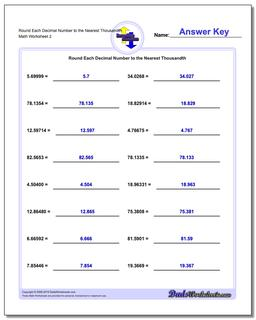PLEASE GO BACK AND USE THE BIG BLUE 'PRINT' BUTTON ON THE PAGE TO PRINT THE WORKSHEET CORRECTLY!Sorry for the trouble! The browser won't print the embedded worksheet PDF directly using the normal 'Print' command in the file menu, so you need to click the big 'Print' button to send just the worksheet and not the surrounding page to the printer.

# Math Worksheets: Rounding Numbers: Rounding Numbers: Round Each Decimal Number to the Nearest Thousandth (Second Worksheet)## Round Each Decimal Number to the Nearest Thousandth (Second Worksheet)

PropertyValue
DescriptionRound Each Decimal Number to the Nearest Thousandth: Rounding decimal worksheets with decimal numbers where there may be significant digits to the left of the rounding place value. (Second Worksheet)
Resource TypeWorksheet